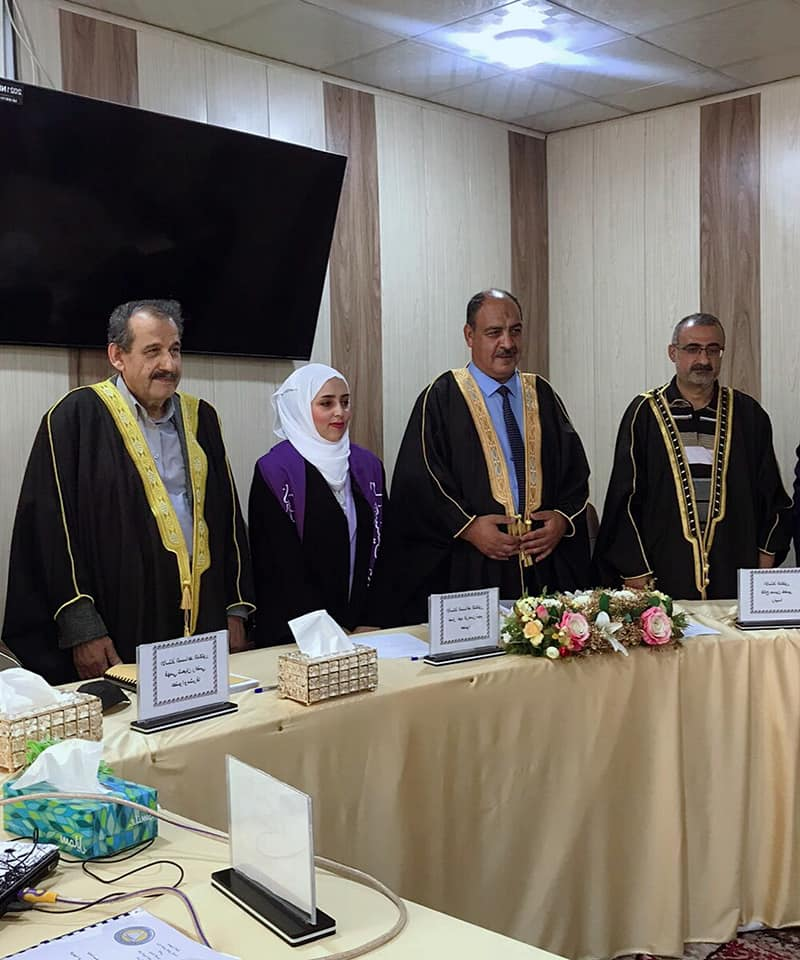## A master's thesis at the University of Basra discusses about (studying energy levels and electromagnetic properties of even-numbered nuclei with N=52 and N=84 within the framework of the model of interacting bosons)

The College of Education for Pure Sciences, Department of Physics, discussed a master's thesis on
Studying the energy levels and electromagnetic properties of even-numbered nuclei with N=52 and N=84 within the framework of the model of interacting bosons.
The message presented by the researcher (Russell Zaki Salman) included
A study of the electric quadrupole moment and branching ratios and the study of the excited energy levels of the ground beam as a function of the number of protons
The purpose of the message
Calculating the positively symmetric excited energy levels, as well as calculating the electromagnetic quadrupole transition probability B(E2) and the magnetic dipole transition probability B(M1) and electromagnetic mixing ratios. The mixed levels of symmetry were studied using the IBM-2 model, and the levels were determined
j=1+,2,+,3+ as Mixed Symmetry State MSS
The nuclear potential energy surface was also studied to determine the nuclear shape, and the obtained results were compared with the available practical values, and through good agreement, the model's success in describing the nuclear structure of these isotopes was shown.